Test: Properties of Metals, Simple Stress Strain & Elastic Constants - 1

# Test: Properties of Metals, Simple Stress Strain & Elastic Constants - 1

Test Description

## 10 Questions MCQ Test GATE Civil Engineering (CE) 2023 Mock Test Series | Test: Properties of Metals, Simple Stress Strain & Elastic Constants - 1

Test: Properties of Metals, Simple Stress Strain & Elastic Constants - 1 for Civil Engineering (CE) 2023 is part of GATE Civil Engineering (CE) 2023 Mock Test Series preparation. The Test: Properties of Metals, Simple Stress Strain & Elastic Constants - 1 questions and answers have been prepared according to the Civil Engineering (CE) exam syllabus.The Test: Properties of Metals, Simple Stress Strain & Elastic Constants - 1 MCQs are made for Civil Engineering (CE) 2023 Exam. Find important definitions, questions, notes, meanings, examples, exercises, MCQs and online tests for Test: Properties of Metals, Simple Stress Strain & Elastic Constants - 1 below.
Solutions of Test: Properties of Metals, Simple Stress Strain & Elastic Constants - 1 questions in English are available as part of our GATE Civil Engineering (CE) 2023 Mock Test Series for Civil Engineering (CE) & Test: Properties of Metals, Simple Stress Strain & Elastic Constants - 1 solutions in Hindi for GATE Civil Engineering (CE) 2023 Mock Test Series course. Download more important topics, notes, lectures and mock test series for Civil Engineering (CE) Exam by signing up for free. Attempt Test: Properties of Metals, Simple Stress Strain & Elastic Constants - 1 | 10 questions in 30 minutes | Mock test for Civil Engineering (CE) preparation | Free important questions MCQ to study GATE Civil Engineering (CE) 2023 Mock Test Series for Civil Engineering (CE) Exam | Download free PDF with solutions
 1 Crore+ students have signed up on EduRev. Have you?
Test: Properties of Metals, Simple Stress Strain & Elastic Constants - 1 - Question 1

### A material has identical properties in all directions, it is said to be

Detailed Solution for Test: Properties of Metals, Simple Stress Strain & Elastic Constants - 1 - Question 1

Isotropic- Identical properties in all directions
Orthotropic- Different properties in all three directions
Homogeneous- A material of uniform composition

Test: Properties of Metals, Simple Stress Strain & Elastic Constants - 1 - Question 2

### The term nominal stress in stress-strain curve for mild steel implies

Detailed Solution for Test: Properties of Metals, Simple Stress Strain & Elastic Constants - 1 - Question 2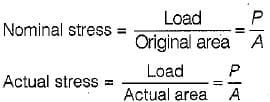Actual area at instant of loading does not remain constant and decreases with increases in elongation actual stress is also called true stress.

Test: Properties of Metals, Simple Stress Strain & Elastic Constants - 1 - Question 3

### For metallic minerals creep becomes an important consideration at

Detailed Solution for Test: Properties of Metals, Simple Stress Strain & Elastic Constants - 1 - Question 3

The temperature at which the creep becomes an important consideration is called HOMOLOGOUS TEMPERATURE and this temperature is nearly half of the melting point temperature.

Test: Properties of Metals, Simple Stress Strain & Elastic Constants - 1 - Question 4

Clapeyron's theorem is associated with the analysis of

Detailed Solution for Test: Properties of Metals, Simple Stress Strain & Elastic Constants - 1 - Question 4

Clapeyorn’s Theorem of Three Moments express the relation b/w bending moments at three successive supports of a continuous beam, subjected to a loading on two adjacent span with or without settlement of supports.

Test: Properties of Metals, Simple Stress Strain & Elastic Constants - 1 - Question 5

A rubber band is elongated to double its initial length, its true strain is

Detailed Solution for Test: Properties of Metals, Simple Stress Strain & Elastic Constants - 1 - Question 5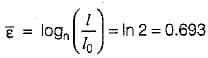Test: Properties of Metals, Simple Stress Strain & Elastic Constants - 1 - Question 6

A prismatic beam has uniform

Detailed Solution for Test: Properties of Metals, Simple Stress Strain & Elastic Constants - 1 - Question 6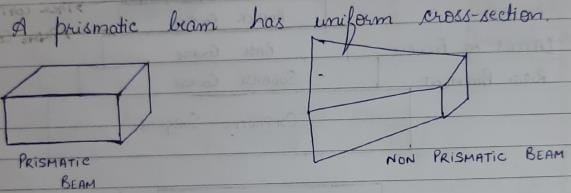Test: Properties of Metals, Simple Stress Strain & Elastic Constants - 1 - Question 7

In the case of pure bending, the beam will bend into an arc of a

Detailed Solution for Test: Properties of Metals, Simple Stress Strain & Elastic Constants - 1 - Question 7

The equation for simple bending is given by: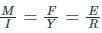where I = Moment of Inertia, E = Modulus of Elasticity, F = Stress at any fiber at a distance of y from the neutral axis, M = Bending moment and R = Radius of curvature.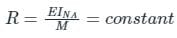For a circle, the radius is constant, hence for pure bending, the arc bend into a circle.

Assumptions made in the theory of Pure Bending :

• The material of the beam is homogeneous and isotropic
• The value of Young's Modulus of Elasticity is the same in tension and compression
• The transverse sections which were plane before bending, remain plane after bending also
• The beam is initially straight and all longitudinal filaments bend into circular arcs with a common centre of curvature
• The radius of curvature is large as compared to the dimensions of the cross-section
• Each layer of the beam is free to expand or contract, independently of the layer, above or below it.
Test: Properties of Metals, Simple Stress Strain & Elastic Constants - 1 - Question 8

If the modulus of elasticity is zero, the material is said to be

Detailed Solution for Test: Properties of Metals, Simple Stress Strain & Elastic Constants - 1 - Question 8

E =0  Slope of Stress- Strain Curve is straight horizontal line. It means at constant stress, strain keeps increasing which is the characteristic property of perfectly plastic material.

Test: Properties of Metals, Simple Stress Strain & Elastic Constants - 1 - Question 9

The compressibility of a material is proportional to

Detailed Solution for Test: Properties of Metals, Simple Stress Strain & Elastic Constants - 1 - Question 9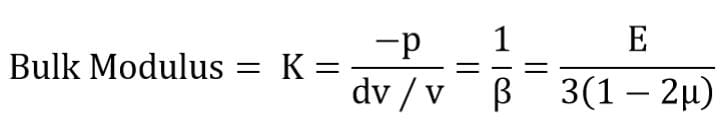Bulk Modulus= K=(-p)/(ⅆv∕v)=1/β=E/3(1-2μ)

β=compressibility is proportional to reciprocal of E.

Test: Properties of Metals, Simple Stress Strain & Elastic Constants - 1 - Question 10

If a beam with the rectangular cross-section is obtained by cutting from circular log of timber, then for the beam to have strongest section in bending, the ratio of breadth to depth should be

Detailed Solution for Test: Properties of Metals, Simple Stress Strain & Elastic Constants - 1 - Question 10

AB = b = D cosθ, BC = d = D sinθ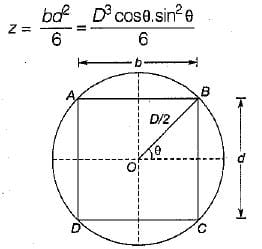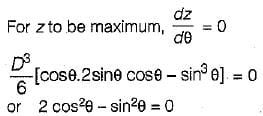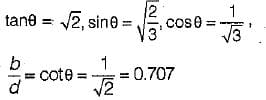## GATE Civil Engineering (CE) 2023 Mock Test Series

27 docs|296 tests
 Use Code STAYHOME200 and get INR 200 additional OFF Use Coupon Code
Information about Test: Properties of Metals, Simple Stress Strain & Elastic Constants - 1 Page
In this test you can find the Exam questions for Test: Properties of Metals, Simple Stress Strain & Elastic Constants - 1 solved & explained in the simplest way possible. Besides giving Questions and answers for Test: Properties of Metals, Simple Stress Strain & Elastic Constants - 1, EduRev gives you an ample number of Online tests for practice

## GATE Civil Engineering (CE) 2023 Mock Test Series

27 docs|296 tests